# Android OpenGLES2.0（十八）——轻松搞定Blend颜色混合

Blend是OpenGL中的一个非常重要的部分，它可以让每个输出的源和目的颜色以多种方式组合在一起，以呈现出不同的效果，满足不同的需求。

## Blend相关函数及意义

//调用此方法，传入GL_BLEND开启BLEND功能
void glEnable(GLenum cap);
//调用此方法，出入GL_BLEND关闭BLEND功能
void glDisable(GLenum cap);
//设置BLEND颜色，结合glBlendFuncSeparate或glBlendFunc使用
void glBlendColor(GLclampf red,GLclampf green,GLclampf blue,GLclampf alpha);
//设置BLEND方程式
void glBlendEquation(GLenum mode);
//对RGB和Alpha分别设置BLEND方程式
void glBlendEquationSeparate(GLenum modeRGB,GLenum modeAlpha);
//设置BLEND函数
void glBlendFunc(GLenum sfactor,GLenum dfactor);
//对RGB和Alpha分别设置BLEND函数
void glBlendFuncSeparate(GLenum srcRGB,GLenum dstRGB,GLenum srcAlpha,GLenum dstAlpha);

Blend的使用比较简单，但是如果不理解Blend的这些函数及参数的意义，使用了错误的参数，就难以获得我们所期望的混合结果了。

glBlendFuncglBlendFuncSeparate都是设置混合因子，反正就是这么个意思了。区别在于glBlendFunc是设置RGBA的混合因子，而glBlendFuncSeparate是分别设置RGB和Alpha的混合因子。设置混合因子是做什么的呢？继续看。
glBlendEquationglBlendEquationSeparate都是设置Blend的方程式，也就是设置混合的计算方式了，具体参数后面说。他们的区别在同glBlendFuncglBlendFuncSeparate的区别一样。

## glBlendFunc及glBlendFuncSeparate详细说明

glBlendFuncSeparate设置混合因子，参数及它们表示的主要如下，而glBlendFunc的参数也是这些，表示的意义就是RGB和A合并为RGBA就是了。在下表中，s0表示源，d表示目的，c表示有glBlendColor设置进来的常量。

ParameterRGB FactorAlpha Factor
GL_ZERO(0, 0, 0)0
GL_ONE(1, 1, 1)1
GL_SRC_COLOR(Rs0, Gs0, Bs0)As0
GL_ONE_MINUS_SRC_COLOR(1, 1, 1) - (Rs0, Gs0, Bs0)1 - As0
GL_ONE_MINUS_DST_COLOR(1, 1, 1) - (Rd, Gd, Bd)1 - Ad
GL_SRC_ALPHA(As0, As0, As0)As0
GL_ONE_MINUS_SRC_ALPHA(1, 1, 1) - (As0, As0, As0)1 - As0
GL_CONSTANT_COLOR(Rc, Gc, Bc)Ac
GL_ONE_MINUS_CONSTANT_COLOR(1, 1, 1) - (Rc, Gc, Bc)1 - Ac
GL_CONSTANT_ALPHA(Ac, Ac, Ac)Ac
GL_ONE_MINUS_CONSTANT_ALPHA(1, 1, 1) - (Ac, Ac, Ac)1 - Ac
GL_SRC_ALPHA_SATURATE(i, i, i)1

## glBlendEquation及glBlendEquationSeparate详细说明

glBlendEquationSeparate的设置混合操作，参数及其意义如下表所示。通过glBlendEquationSeparate或者glBlendEquation设置的方程中，源和目的颜色分别为 (Rs,Gs,Bs,As) $(R_s, G_s, B_s, A_s)$ (Rd,Gd,Bd,Ad) $(R_d, G_d, B_d, A_d)$。最终混合的颜色结果为 (Rr,Gr,Br,Ar) $(R_r, G_r, B_r, A_r)$。源和目的的混合因子分别为 (sR,sG,sB,sA) $(s_R, s_G, s_B, s_A)$ (dR,dG,dB,dA) $(d_R, d_G, d_B, d_A)$。其中，所有的颜色分量的取值范围都为[ 0, 1 ]。GL_MIN和GL_MAX是在OpenGLES3.0才有的

ModeRGB ComponentsAlpha Component
GL_FUNC_ADD RrGrBr=sRRs+dRRd=sGGs+dGGd=sBBs+dBBd \begin{align}R_r & = s_R R_s + d_R R_d\\G_r & = s_G G_s + d_G G_d\\B_r & = s_B B_s + d_B B_d\end{align} Ar=sAAs+dAAd $A_r = s_A A_s + d_A A_d$
GL_FUNC_SUBTRACT RrGrBr=sRRsdRRd=sGGsdGGd=sBBsdBBd \begin{align}R_r & = s_R R_s - d_R R_d\\G_r & = s_G G_s - d_G G_d\\B_r & = s_B B_s - d_B B_d\end{align} Ar=sAAsdAAd $A_r = s_A A_s - d_A A_d$
GL_FUNC_REVERSE_SUBTRACT RrGrBr=dRRdsRRs=dGGdsGGs=dBBdsBBs \begin{align}R_r & = d_R R_d - s_R R_s\\G_r & = d_G G_d - s_G G_s\\B_r & = d_B B_d - s_B B_s\end{align} Ar=dAAdsAAs $A_r = d_A A_d - s_A A_s$
GL_MIN RrGrBr=min(Rs,Rd)=min(Gs,Gd)=min(Bs,Bd) \begin{align}R_r & = min(R_s, R_d)\\G_r & = min(G_s, G_d)\\B_r & = min(B_s, B_d)\end{align} Ar=min(As,Ad) $A_r = min(A_s, A_d)$
GL_MAX RrGrBr=max(Rs,Rd)=max(Gs,Gd)=max(Bs,Bd) \begin{align}R_r & = max(R_s, R_d)\\G_r & = max(G_s, G_d)\\B_r & = max(B_s, B_d)\end{align} Ar=max(As,Ad) $A_r = max(A_s, A_d)$

## Blend代码示例

@Override
public void onSurfaceCreated(GL10 gl, EGLConfig config) {
GLES20.glClearColor(0,0,0,0);
mSrcFilter.create();
mDstFilter.create();
int[] textures=new int;

//导入一张图片设置为源纹理
GLES20.glGenTextures(2,textures,0);
GLES20.glBindTexture(GLES20.GL_TEXTURE_2D,textures);
EasyGlUtils.useTexParameter();
GLUtils.texImage2D(GLES20.GL_TEXTURE_2D,0,GLES20.GL_RGBA,srcBitmap,0);
mSrcFilter.setTextureId(textures);
//再导入一张图片设置为目标纹理
GLES20.glBindTexture(GLES20.GL_TEXTURE_2D,textures);
EasyGlUtils.useTexParameter();
GLUtils.texImage2D(GLES20.GL_TEXTURE_2D,0,GLES20.GL_RGBA,dstBitmap,0);
mDstFilter.setTextureId(textures);

}

@Override
public void onSurfaceChanged(GL10 gl, int width, int height) {
this.width=width;
this.height=height;
mSrcFilter.setSize(width,height);
mDstFilter.setSize(width,height);
MatrixUtils.getMatrix(mDstFilter.getMatrix(),MatrixUtils.TYPE_FITSTART,
dstBitmap.getWidth(),dstBitmap.getHeight(),width,height);
MatrixUtils.getMatrix(mSrcFilter.getMatrix(),MatrixUtils.TYPE_FITSTART,
srcBitmap.getWidth(),srcBitmap.getHeight(),width,height);
}

@Override
public void onDrawFrame(GL10 gl) {
GLES20.glClear(GLES20.GL_COLOR_BUFFER_BIT | GLES20.GL_DEPTH_BUFFER_BIT);
//开启Blend
GLES20.glEnable(GLES20.GL_BLEND);
//设置BlendFunc，第一个参数为源混合因子，第二个参数为目的混合因子
GLES20.glBlendFunc(nSrcPar,nDstPar);
//设置BlendEquation，GLES2.0中有三种
GLES20.glBlendEquation(equaInt[nEquaIndex]);
GLES20.glViewport(0,0,width,height);
//先渲染目的纹理出来，再渲染源纹理出来，是源纹理去与目的纹理混合
mDstFilter.draw();
mSrcFilter.draw();
}1. 当目标和源因子都设置为GL_ZERO，无论混合方程怎样设置，最终肯定啥也没有。
2. 当源设置为GL_ONE，目标设置为GL_ZERO，方程设置为加还是减，最终应该渲染的就是目标的颜色，也就是之渲染出金币。
3. 当源设置为GL_ONE，目标设置为GL_SRC_COLOR，方程设置为加，根据公式最终颜色=(目标颜色*目标因子)+(源颜色*源因子)，得到最终有颜色的区域必定是源alpha不为0的区域，因为源是作为目标因子的，源*目标，最终源中alpha为0的区域，这个结果也为0，也就是最终的结果区域透明了。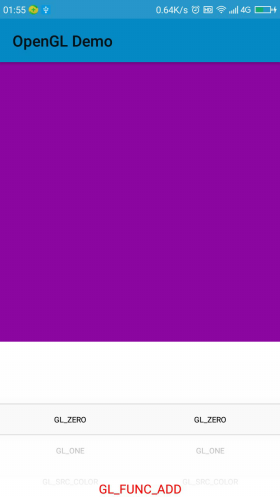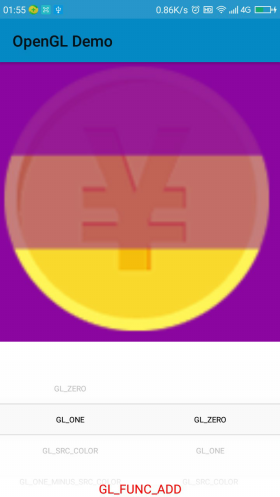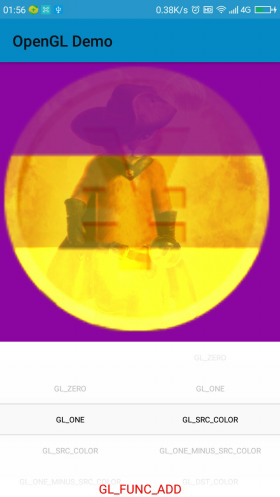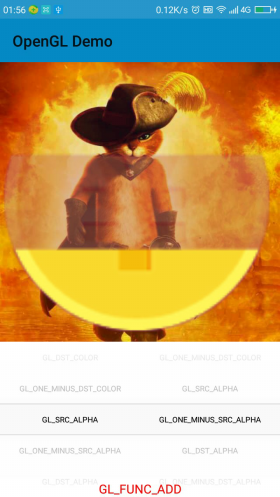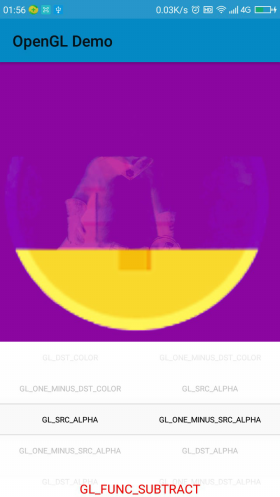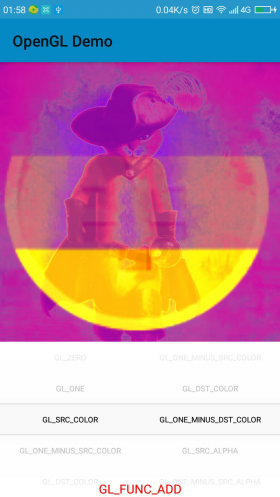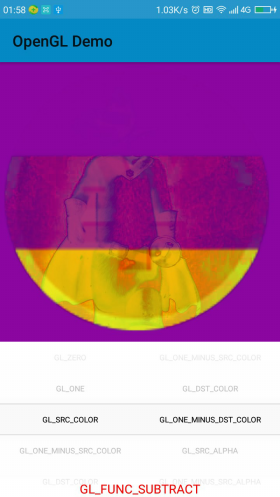## 源码

06-122万+05-234896
08-151372
02-1149
11-16671
08-29849
11-262962
12-091万+